# T-SQL SET Operators Part 2: INTERSECT and EXCEPT

In my previous article, I explained the basics of set operators, their types, and prerequisites for their use. I also talked about UNION and UNION ALL operators, their usage and differences.

In this article, we’re going to learn the following:

1. EXCEPT and INTERSECT operators.
2. Difference between INTERSECT and INNER JOIN.
3. The detailed explanation of INTERSECT and EXCEPT with an example.

EXCEPT and INTERSECT operators were introduced in SQL Server 2005. Both are set operators used to combine the result sets generated by two queries and retrieve the desired output.

### What is the INTERSECT operator

INTERSECT is used to get records common to all data sets retrieved from multiple queries or tables. Here’s a visualization of this: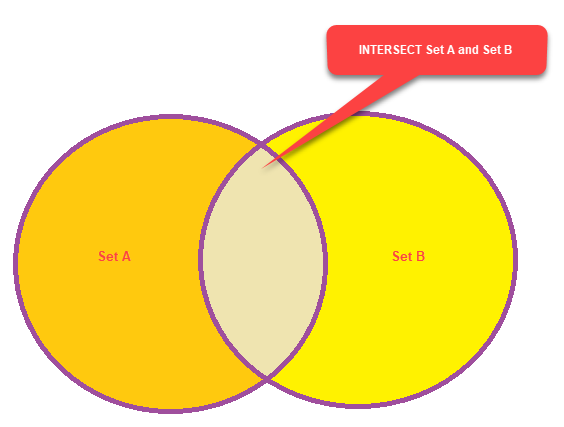The syntax of the INTERSECT operator is a follows:

### What is the EXCEPT operator

EXCEPT is used to retrieve records which are found in one query but not in another query. In other words, it returns records which are unique to one result set. This is what it looks like visualized: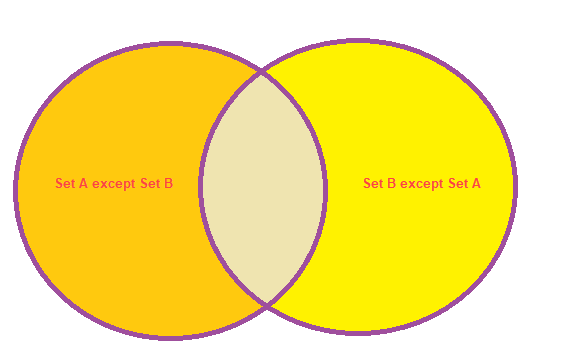The syntax of the EXCEPT operator is as follows:

Let’s create a demo setup to demonstrate how these operators can be used.

### Demo Setup

To demonstrate INTERSECT and EXCEPT, I created two tables named Employee and Trainee.

Execute the following query to create these tables:

Now, let’s insert some dummy data into the Employee table by executing the following query:
Next, we’ll do the same thing for the Trainee table:
Now, let’s use INTERSECT to retrieve the list of employees which are common to both tables. To do that, run the following query:
The output of this query should be as follows: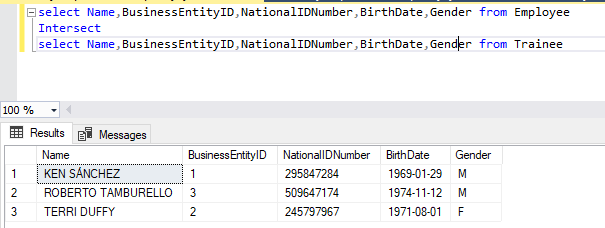As you can see in the screenshot above, the query has only returned records which are common to both tables.

### INNER JOIN vs. INTERSECT

In most cases, INTERSECT and INNER JOIN return the same output, but there are some exceptions. A simple example will help us understand this.

Let’s add some duplicate records to the Trainee table. Execute the following query:

Now, we’ll try to generate the desired output using INTERSECT.

This is the output we get:Now, let’s try using INNER JOIN.

The output we get in this case is as follows: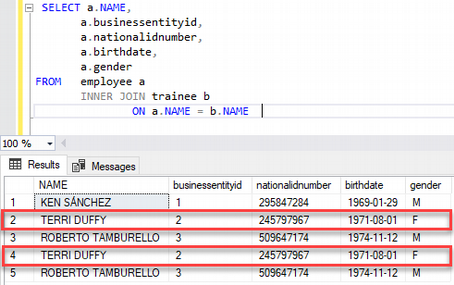Now, as you can see on the screenshot above, INNER JOIN retrieves records which are common to both tables. It populates all records from the right table. Therefore, you can see duplicate records.

Now, let’s add the DISTINCT keyword to the INNER JOIN query and look at what this does:

The output should look like this: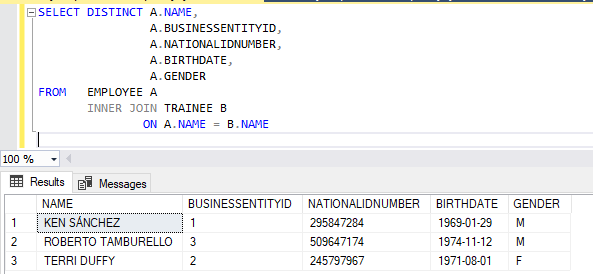As you can see on the screenshot above, duplicate records have been eliminated.

INTERSECT and INNER JOIN treat NULL values differently. For INNER JOIN, two NULL values are different, so there are chances that it will skip them while joining two tables.

On the other hand, INTERSECT treats two NULL values as being the same, so records that have NULL values won’t be eliminated. To understand it better, let’s look at an example.

First, let’s add some NULL values to the Trainee and Employee tables by executing the following query:

Now let’s try to retrieve records common to the two tables using INTERSECT and INNER JOIN. You will need to execute the following query:

This is the output we should get as a result: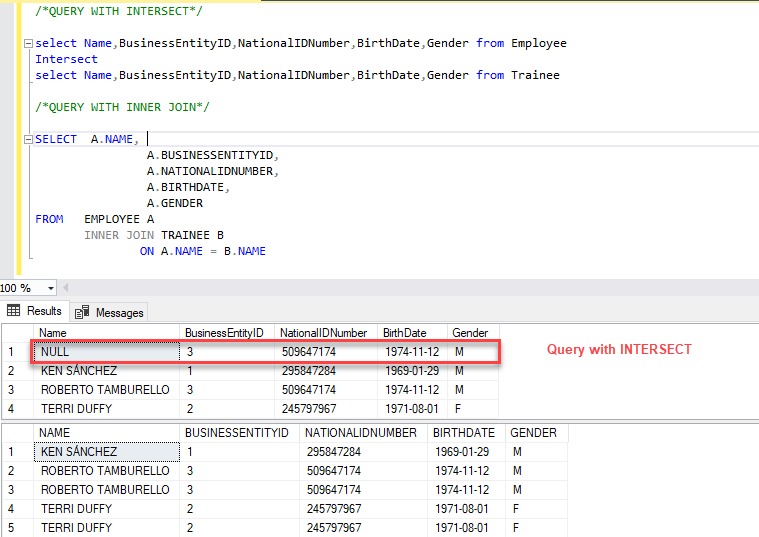As you can see above, the result set generated by INTERSECT contains NULL values, while INNER JOIN skipped the records that have NULL values.

### The EXCEPT Operator

To demonstrate the EXCEPT operator in action, let’s look at a use case. For example, I want to populate the details of female employees from the Employee table. The following query will help us do just that:

This is the output we get: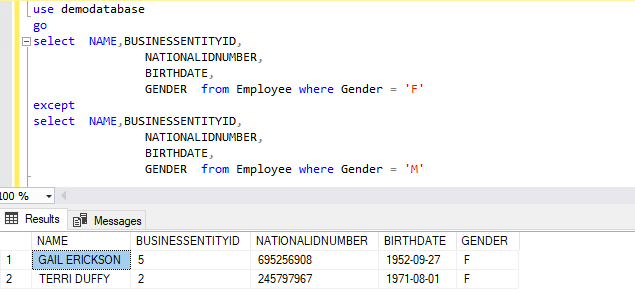As you can see above, the query populated only the female employees’ details.

You can also populate the result set using a sub-query:

### Limitations of INTERSECT and EXCEPT

1. We cannot use EXCEPT and INTERSECT in distributed partitioned view definitions with COMPUTE and COMPUTE BY clauses.
2. EXCEPT and INTERSECT can be used in Fast forward-only and static cursors.
3. EXCEPT and INTERSECT can be used in distributed queries, but can only be executed on the local server. You cannot run them on a remote server.

### Summary